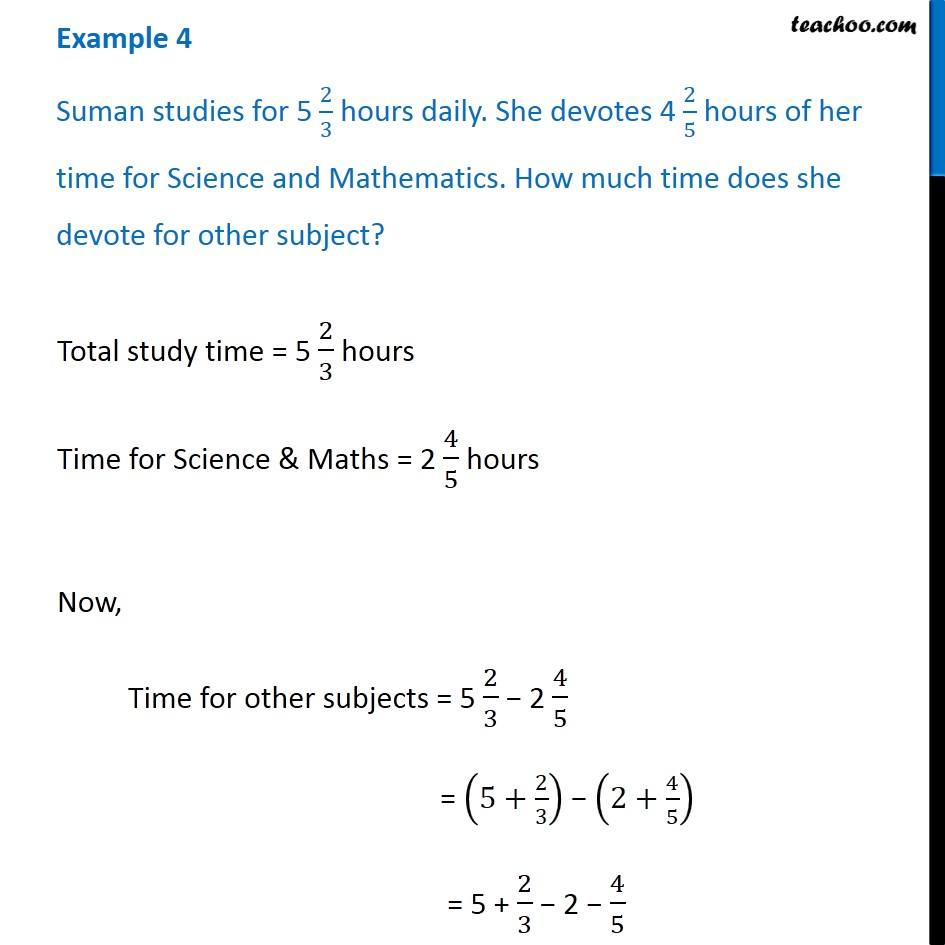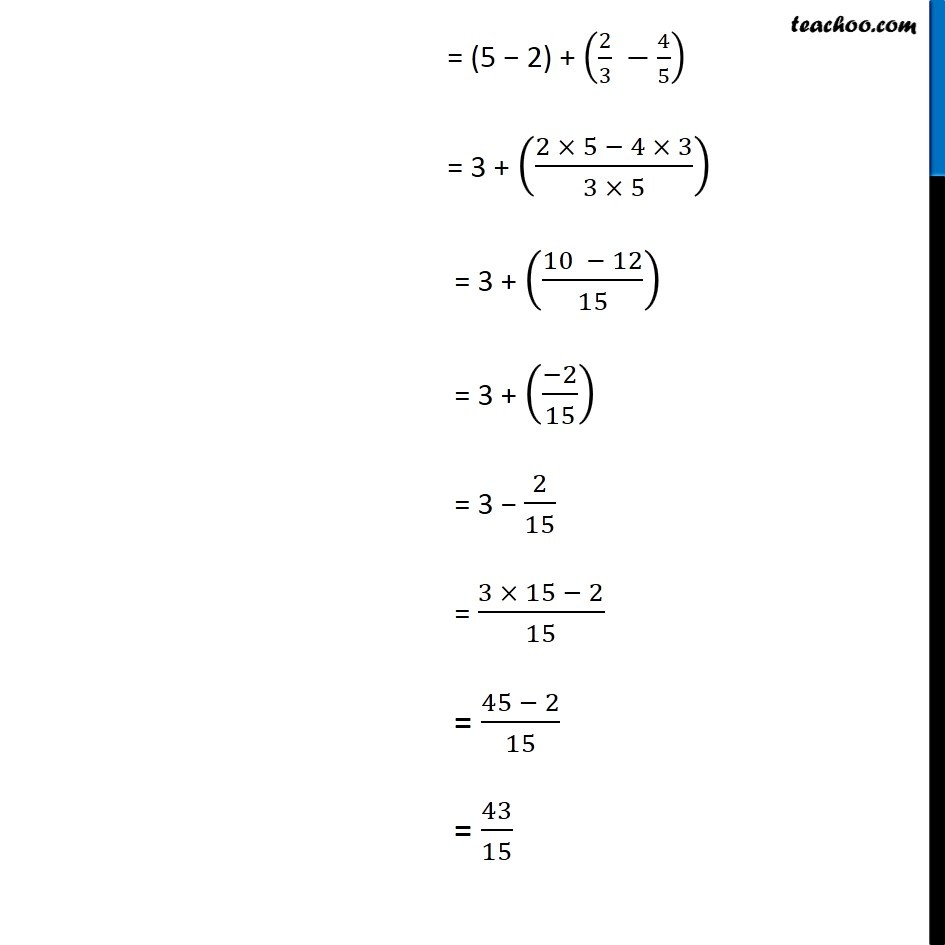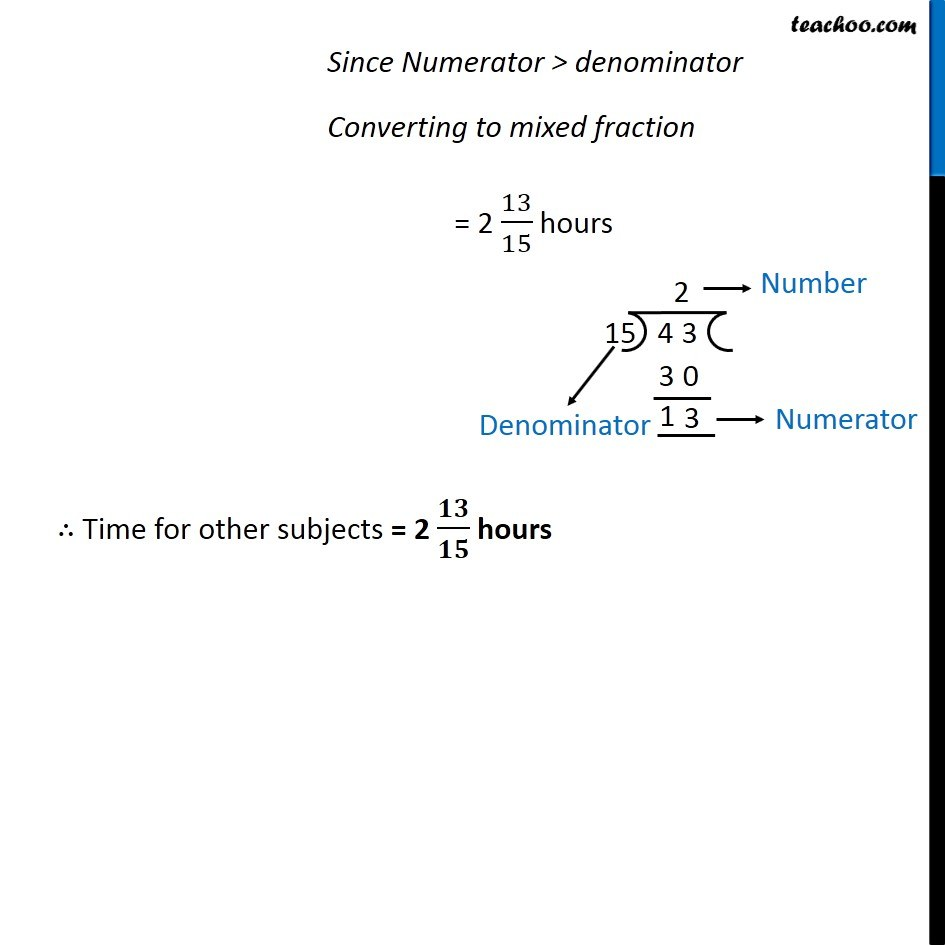Addition and Subtraction of Fractions - Statement Questions

Chapter 2 Class 7 Fractions and Decimals
Concept wise### Transcript

Example 4 Suman studies for 5 2/3 hours daily. She devotes 4 2/5 hours of her time for Science and Mathematics. How much time does she devote for other subject?Total study time = 5 2/3 hours Time for Science & Maths = 2 4/5 hours Now, Time for other subjects = 5 2/3 − 2 4/5 = (5+2/3) − (2+4/5) = 5 + 2/3 − 2 − 4/5 = (5 − 2) + (2/3 −4/5) = 3 + ((2 × 5 − 4 × 3)/(3 × 5)) = 3 + ((10 − 12)/15) = 3 + ((−2)/15) = 3 − 2/15 = (3 × 15 − 2)/15 = (45 − 2)/15 = 43/15 Since Numerator > denominator Converting to mixed fraction = 2 13/15 hours ∴ Time for other subjects = 2 𝟏𝟑/𝟏𝟓 hours